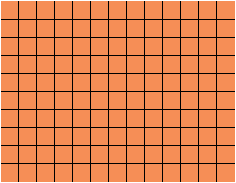Name: Marlene Who is asking: Other Level of the question: All Question: want to convert 13ft by 10ft room area into square feet, have no idea how to do the math. thanks Hi Marlene, If you think about putting tile on the floor using tiles that are 1 foot by 1 foot, that is one square foot each then you would have 10 rows of 13 tiles each.This would then require 1013 = 130 tiles which is 130 square feet. Penny